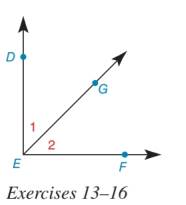Chapter 1.5, Problem 16E### Elementary Geometry for College St...

6th Edition
Daniel C. Alexander + 1 other
ISBN: 9781285195698

#### Solutions

Chapter
Section### Elementary Geometry for College St...

6th Edition
Daniel C. Alexander + 1 other
ISBN: 9781285195698
Textbook Problem
1 views

# In Exercises 11 to 22, use the Given information to draw a conclusion based on the stated property or definition.Given: m ∠ 1 + m ∠ 2 = 90 ° ; definition of complementary angles.

To determine

To find:

The conclusion based on the stated property.

Explanation

Given:

The given diagram is,

Given: m1+m2=90°; definition of complementary angles.

Definition:

If the sum of two angles is 90°, then the angles are known as complementary angles. Each angle in the pair is the complement of the other angle

### Still sussing out bartleby?

Check out a sample textbook solution.

See a sample solution

#### The Solution to Your Study Problems

Bartleby provides explanations to thousands of textbook problems written by our experts, many with advanced degrees!

Get Started

#### Find more solutions based on key concepts